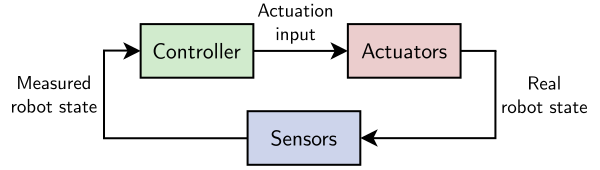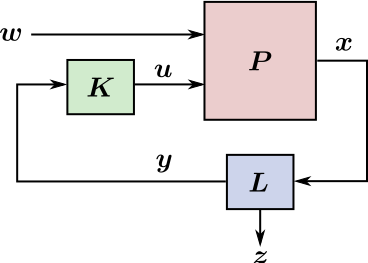# What is a controller?

Robots run many layers of software. The layer that decides what actions to take based on the latest sensor readings is called the controller. In reinforcement learning, it is known as the agent.

## Feedback loop

In the general sense, robot components can be classified in three categories: actuation (“the plant”, sometimes simply called “the robot”), sensing and control. A controller is, by definition,anything that turns robot outputs (a.k.a. “states”, for instance joint positions and velocities) into new robot inputs (a.k.a. “controls”, for instance joint accelerations or torques). Robot outputs are not known perfectly but measured by sensors. Putting these three components together yields the feedback loop:If we take the simple example of a velocity-controlled point mass, the input of your robot is a velocity and its output is a new position. A controller is then any piece of software that takes positions as inputs and outputs velocities. Under the hood, the controller may carry out various operations such as trajectory planning or PID feedback. For instance, a model predictive controller computes a desired future trajectory of the robot starting from its current (measured) state, then extracts the first controls from it and sends it to the robot.

### Example of HRP-4

The HRP-4 humanoid robot from Kawada Industries is a position-controlled robot.

• Inputs: desired joint positions
• Outputs: joint angle positions, as well as the position and orientation of the robot with respect to the inertial frame (a.k.a. its floating base transform)

Being a mobile robot, it is underactuated, meaning its state has a higher dimension than its inputs. Measurements of the robot state are carried out by rotary encoders for joint angles and using an IMU for the free-flyer transform. A controller for HRP-4 therefore takes as inputs the robot’s state (joint positions + position and orientation with respect to the inertial frame) and outputs a new set of desired positions.

## Terminology

Control theory has a bit more terminology to describe the feedback loop above. Here is the same loop with standard notations from this field:The real state $$\bfx$$ of the system is an ideal quantity. Some components of it may not be observable by sensors, for example the yaw orientation of the robot with respect to gravity.

• $$\bfy$$ is the output, the set of observable components in $$\bfx$$.
• $$\bfz$$ is the exogenous output, the set of non-observable components in $$\bfx$$.
• $$\bfL$$ is the state observer that estimates $$\bfy$$ from available sensor readings.
• $$\bfK$$ is the controller that computes the control input from the measured state.
• $$\bfu$$ is the control input by which the controller can act on the plant.
• $$\bfP$$ is the plant, the external system being controlled, a.k.a. the robot.
• $$\bfw$$ is the exogenous input, a set of external causes unseen by the controller.

The vocabulary of control theory puts the plant-observer at the center, which is why $$\bfu$$ is called the control input (although it's an output of the controller: because it's an input to the plant) and $$\bfy$$ is called the output (it's an output of the observer and an input to the controller).

### Exogenous inputs

Control theory precises the notion of exogenous inputs $$\bfw$$ to represent external causes that the system cannot observe. For example, when a humanoid robot $$\bfP$$ walking on unknown terrain is subjected to an external push $$\bfw$$, the robot cannot distinguish a terrain irregularity (there is a difference between the expected and measured contact forces caused by some unknown terrain shape) from the external push (the contact force difference is caused by the push). The push is then an exogenous input, required to integrate $$\bfP$$ forward in time but unknown to the controller $$\bfK$$.

### Reinforcement learning

Reinforcement learning frames the same problem with a slightly different terminology. In reinforcement learning, $$\bfy$$ is the observation, $$\bfu$$ is the action, $$\bfP$$ is the environment and $$\bfK$$ is the agent. Most of the time, exogenous quantiies and the state observer $$\bfL$$ are collectively included in $$\bfP$$, as reinforcement learning centers on the agent. The addition of reinforcement learning to classical control theory is a second input $$r$$ to the controller representing the reward obtained from executing action $$\bfu$$ from state $$\bfx$$.

## Q & A

How to achieve velocity/acceleration control on a position-controlled robot?

Sending successive joint angles along a trajectory with the desired velocities or accelerations, and relying and the robot’s stiff position tracking.

How to control the underactuated position of the robot in the inertial frame?

Using contacts with the environment and force control. For instance, position-controlled robots use admittance control to regulate forces at their end-effectors.

## To go further

There are many references to pick from in control theory. For an introduction, you can check out Quang-Cuong Pham’s lecture notes on control theory.